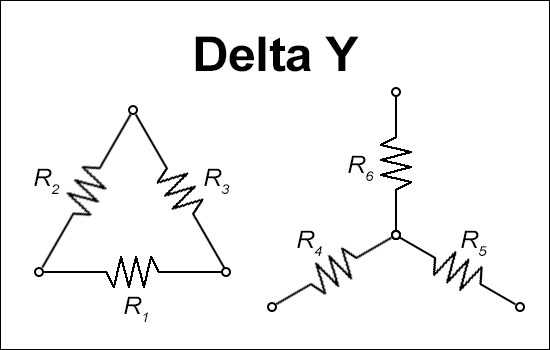Deprecated: The behavior of unparenthesized expressions containing both '.' and '+'/'-' will change in PHP 8: '+'/'-' will take a higher precedence in /home/sonota/trance-cat.com/public_html/electrical-circuit-calculators/en/delta-y-conversion-calculator.php on line 109

Deprecated: The behavior of unparenthesized expressions containing both '.' and '+'/'-' will change in PHP 8: '+'/'-' will take a higher precedence in /home/sonota/trance-cat.com/public_html/electrical-circuit-calculators/en/delta-y-conversion-calculator.php on line 113
Delta Y Conversion Calculator

# Delta Y Conversion Calculator

Output values are rounded to the second decimal place.### Input

R1/R4:

R2/R5:

R3/R6:

Calculate Values for Delta
Calculate Values for Y